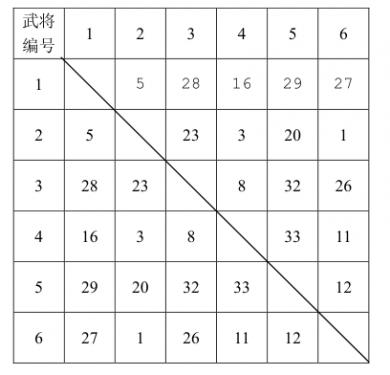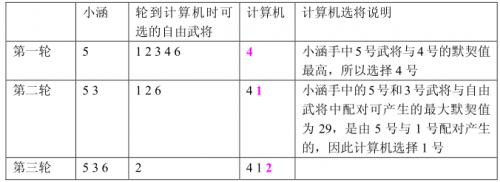# 洛谷P1199 三国游戏

## 题目描述## 输入输出样例

6
5 28 16 29 27
23 3 20 1
8 32 26
33 11
12


1
32



8
42 24 10 29 27 12 58
31 8 16 26 80 6
25 3 36 11 5
33 20 17 13
15 77 9
4 50
19 

1
77

## 说明

【数据范围】

#include <cstdio>
#include <cstring>
#include <iostream>
#include <algorithm>

using namespace std;

int n, d, max1, max2, ans = 0;

int main()
{
scanf("%d", &n);
for (int i = 1; i <= n; i++)
{
for (int j = i + 1; j <= n; j++)
{
scanf("%d", &d[i][j]);
d[j][i] = d[i][j];
}
d[i][i] = 0;
}
for (int i = 1; i <= n; i++)
{
max1 = max2 = 0;
for (int j = 1; j <= n;j++)
if (d[i][j] > max1)
{
max2 = max1;
max1 = d[i][j];
}
else
if (d[i][j] > max2)
max2 = d[i][j];
if (max2 > ans)
ans = max2;
}
printf("1\n%d\n", ans);

return 0;
}

posted @ 2017-09-13 22:03 zbtrs 阅读(...) 评论(...) 编辑 收藏